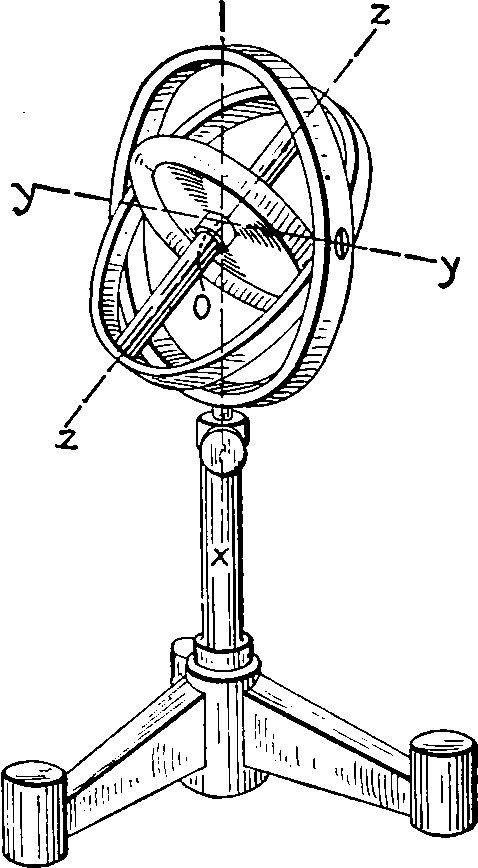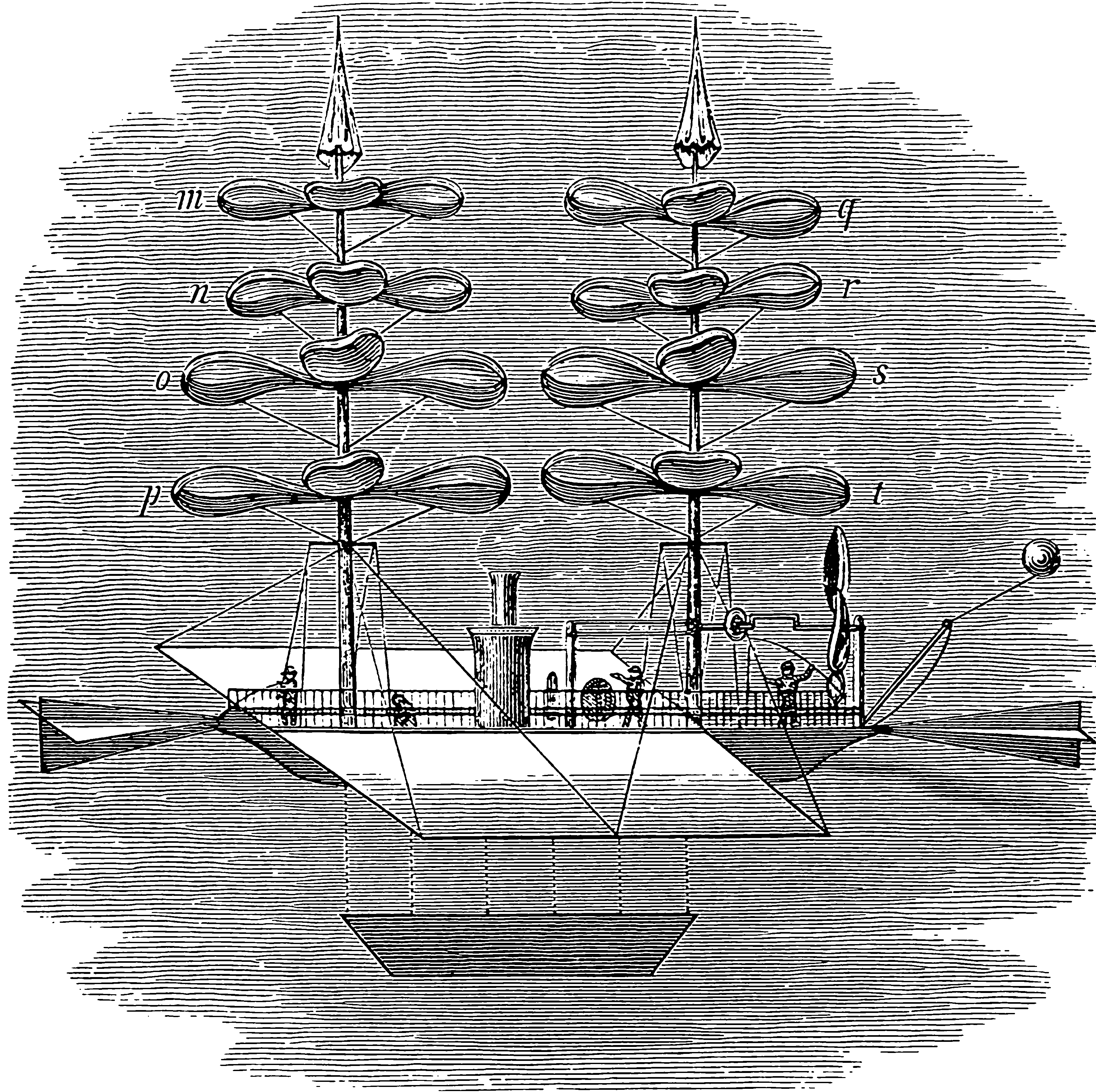# Orthonormal and unitary matrices

## Energy preserving operators, generalized rotations

(

In which I think about parameterisations and implementations of finite dimensional energy-preserving operators, a.k.a. matrices. A particular nook in the linear feedback process library, closely related to stability in linear dynamical systems, since every orthonormal matrix is the forward operator of an energy-preserving system, which is an edge case for certain natural types of stability. Also important in random low-dimensional projections.Uses include maintaining stable gradients in recurrent neural networks and efficient invertible normalising flows . Also, parameterising stable Multi-Input-Multi-Output (MIMO) delay networks in signal processing.

There is some terminological work to be done. Some writers refer to orthogonal matrices (but I prefer that to mean matrices where the columns are not necessarily length 1), and some refer to unitary matrices, which seems to imply the matrix is over the complex field instead of the reals but is basically the same from my perspective.

We also might want to consider the implied manifolds upon which these objects live, the Stiefel manifold. Formally, the Stiefel manifold $$\mathcal{V}_{k, m}$$ is the space of $$k$$ frames in the $$m$$ -dimensional real Euclidean space $$\mathbb{R}^{m},$$ represented by the set of $$m \times k$$ matrices $$\mathrm{M}$$ such that $$\mathrm{M}^{\prime} \mathrm{M}=\mathrm{I}_{k},$$ where $$\mathrm{I}_{k}$$ is the $$k \times k$$ identity matrix. Usually my purposes are served here by $$k=m$$. There are some interesting cases in low dimensional projections served by $$k<m,$$ including $$k=1.$$

Finding an orthonormal matrix is equivalent to choosing a finite orthonormal basis, so any way we can parameterise such a basis gives us an orthonormal matrix.

NB the normalisation implies that the basis for an $$n\times n$$ matrix has a most $$n(n-1)$$ free parameters.

## Take the QR decomposition

HT Russell Tsuchida for pointing out that the $$\mathrm{Q}$$ matrix in the QR decomposition, $$\mathrm{M}=\mathrm{Q}\mathrm{R}$$ by construction gives me an orthonormal matrix from any square matrix. Likewise with the $$\mathrm{U},\mathrm{V}$$ matrices in the $$\mathrm{M}=\mathrm{U}\Sigma \mathrm{V}^*$$ SVD. This construction is overparameterised, with $$n^2$$ free parameters.

The construction of the QR decomposition Householder reflections is, Wikipedia reckons, $$\mathcal{O}(n^3)$$ multiplications for an $$n\times n$$ matrix.

We can, however, also use the Lanczos algorithm to find an orthonormal basis for a matrix which admits of warm restarts, although the middle matrix is tri-diagonal which is not quite what we want.

Question do the spectral radius constraints of NO-BEARS upper-bounding the spectral radius, give us a pointer towards another method for finding such matrices? (HT Dario Draca for mentioning this.)

I wonder what the distribution of orthonomal decompositions matrices is for some, say, matrix with independent standard Gaussian entries? Nick Higham has the answer, in his compact introduction to random orthonormal matrices. A uniform, rotation-invariant distribution is given by the Haar measure over the group of orthogonal matrices. He also gives the construction for drawing them by random Householder reflections derived from random standard normal vectors. See random rotations.

## Iterative normalising

Have a nearly orthonormal matrix? Berg et al. (2018) gives a contraction which gets us closer to an orthonormal matrix: $\mathrm{Q}^{(k+1)}=\mathrm{Q}^{(k)}\left(\mathrm{I}+\frac{1}{2}\left(\mathrm{I}-\mathrm{Q}^{(k) \top} \mathrm{Q}^{(k)}\right)\right).$ This reputedly converges if $$\left\|\mathrm{Q}^{(0) \top} \mathrm{Q}^{(0)}-\mathrm{I}\right\|_{2}<1.$$ They attribute this to Björck and Bowie (1971) and Kovarik (1970), wherein it is derived from the Newton iteration for solving $$\mathrm{Q}^{-1}-$$ Here the iterations are clearly $$\mathcal{O}(n^2).$$ An $$\mathcal{O}(n)$$ option would be nice.

## Perturbing an existing orthonormal matrix

Unitary transforms map unitary matrixes to unitary matrixes. We can even start from the identity matrix and perturb it.

### Householder reflections

We can apply successive reflections about hyperplanes, the so called Householder reflections, to an orthonormal matrix to construct a new one. For a unit vector $$v$$ the associated Householder reflection is $\mathrm{H}(v)=\mathrm{I}-2vv^{*}.$ NB $$\det \mathrm{H}=-1$$ so we need to apply an even number of Householder reflections to preserve orthonormality.

### Givens rotationOne obvious method for constructing unitary matrices is composing Givens rotations, which are atomic rotations about 2 axes.

A Givens rotation is represented by a matrix of the form $\mathrm{G}(i,j,\theta )={\begin{bmatrix}1&\cdots &0&\cdots &0&\cdots &0\\\vdots &\ddots &\vdots &&\vdots &&\vdots \\0&\cdots &c&\cdots &-s&\cdots &0\\\vdots &&\vdots &\ddots &\vdots &&\vdots \\0&\cdots &s&\cdots &c&\cdots &0\\\vdots &&\vdots &&\vdots &\ddots &\vdots \\0&\cdots &0&\cdots &0&\cdots &1\end{bmatrix}},}$ where $$c = \cos \theta$$ and $$s = \sim\theta$$ appear at the intersections ith and jth rows and columns. The product $$\mathrm{G}(i,j,\theta)x$$ represents a $$\theta$$-radian counterclockwise rotation of the vector x in the $$(i,j)$$ plane.

## Cayley map

The Cayley map maps the skew-symmetric matrices to the orthogonal matrices of positive determinant, and parameterizing skew-symmetric matrices is easy; just take the upper triangular component of some matrix and flip /negate it. This still requires a matrix inversion in general, AFAICS.

## Exponential map

The exponential map (Golinski et al., 2019). Given a skew-symmetric matrix A, i.e. a $$D \times D$$ matrix such that $$\mathbf{A}^{\top}=-\mathbf{A}$$, the matrix exponential $$\mathbf{Q}=\exp \mathbf{A}$$ is always an orthogonal matrix with determinant 1 . Moreover, any orthogonal matrix with determinant 1 can be written this way. However, computing the matrix exponential takes in general $$\mathcal{O}\left(D^3\right)$$ time, so this parameterization is only suitable for small-dimensional data.

## Parametric sub families

Citing MATLAB, Nick Higham gives the following two parametric families of orthonormal matrices. These are clearly far from covering the whole space of orthonormal matrices.

$q_{ij} = \displaystyle\frac{2}{\sqrt{2n+1}}\sin \left(\displaystyle\frac{2ij\pi}{2n+1}\right)$

$q_{ij} = \sqrt{\displaystyle\frac{2}{n}}\cos \left(\displaystyle\frac{(i-1/2)(j-1/2)\pi}{n} \right)$

Another one: the matrix exponential of a skew-symmetric matrix is orthonormal. If $$\mathrm{A}=-\mathrm{A}^{T}$$ then $\left(e^{\mathrm{A}}\right)^{-1}=\mathrm{e}^{-\mathrm{A}}=\mathrm{e}^{\mathrm{A}^{T}}=\left(\mathrm{e}^{\mathrm{A}}\right)^{T}.$

## Structured

Orthogonal convolutions? TBD

## Random distributions over

See random rotations.

### No comments yet. Why not leave one?

GitHub-flavored Markdown & a sane subset of HTML is supported.